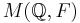# Locally nilpotent not implies normalizer condition

Jump to: navigation, search
This article gives the statement and possibly, proof, of a non-implication relation between two group properties. That is, it states that every group satisfying the first group property (i.e., locally nilpotent group) need not satisfy the second group property (i.e., group satisfying normalizer condition)
View a complete list of group property non-implications | View a complete list of group property implications
Get more facts about locally nilpotent group|Get more facts about group satisfying normalizer condition

## Statement

It is possible to have a group$G$ that is a locally nilpotent group (every finitely generated subgroup of it is a nilpotent group) but is not a group satisfying normalizer condition. In other words,$G$ has a proper subgroup$H$ that is self-normalizing:$H = N_G(H)$.

We can construct examples both for$G$ a torsion-free group and for$G$ a p-group for any prime number$p$.

## Proof

### Description of the example

Take a field$F$. Choose$F$ as follows:

• If we want a torsion-free example, take$F = \mathbb{Q}$, or any other field of characteristic zero.
• If we want an example that is a p-group for some prime number$p$, take$F$ to be the field$\mathbb{F}_p$ of$p$ elements, or alternatively, any field of characteristic$p$.

Let$G$ be McLain's group$M(\mathbb{Q}, F)$.

### Proof that it is locally nilpotent$G$ is locally nilpotent because any finitely generated subgroup of$G$ has finite support in the basis$\mathbb{Q}$, i.e., it moves only finitely many basis elements, so it is in a finite-degree unitriangular matrix group over$F$, which is nilpotent.

### Proof that it does not satisfy the normalizer condition

Let$H$ be the subgroup of$G$ generated by the automorphisms:$e_i \mapsto e_i + re_j, r \in F, i < j \le 0$

and:$e_i \mapsto e_i + re_j, r \in F, 0 < i < j$

Basically, we have partitioned the set$\mathbb{Q}$ into two totally ordered subsets: the non-positive and the positive numbers, and are only considering the automorphisms in$G$ that keep each part within itself.

For an automorphism of the vector space to preserve$H$, it must either preserve both parts of the partition, or interchange them. However, since$G$ is unitriangular, it cannot send anything in the positive part to anything in the non-positive part. Therefore, it cannot interchange the parts, and therefore it must preserve both parts. We can then show that this forces the normalizing element to be within$H$.